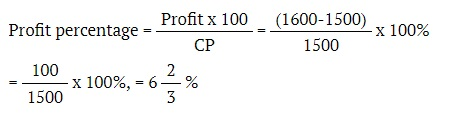## PROFIT AND LOSS

General Rules
Cost Price (CP) : The price at which an article is purchased is called the cost price of the article.
Selling Price (SP) : The price at which an article is sold is called the selling price of the article.
Profit or Gain : If SP is greater than CP, the seller is said to have profit or gain.
Clearly, Profit = (SP)-(CP)
Loss : If SP is less than the cost price, the seller is said to have a loss.
Clearly, Loss = (CP)-(SP)Loss or gain are always reckoned on cost price.Profit on 100 is Profit Percent.Loss on 100 is Loss Percent.
Points to RememberProfit = SP-CPLoss = CP-SP>Profit% = (Profit x 100/CP)Loss% = (Loss x 100/CP)SP = (100 + Profit%/100) x CPSP = (100 - Loss%/100) x CPCP = (100/100 + Profit%) x SPCP = (100/100 = Loss%) x SPIf an article is sold at a Profit of 20% then SP = (120% of CP)If an article is sold at a loss of 20% then SP = (80% of CP)

### Exercise

1. Raju purchased an item for 8200 and sold it at the gain of 25%. From that amount he purchased another item and sold it at the loss of 20%. What is his overall gain/loss?
[a] Loss of 140
[b] Gain of 120
[c] Loss of 150
[d] Neither gain nor loss

In the first case
SP = 125/100 x 8200 = 10250
In the second case
SP = 80/100 x 10250 = 8200
∴ There is neither gain nor loss.

2. A man makes a profit of 10% by selling a radio for 495. What is the cost price of the radio?
[a] 465
[b] 450
[c] 475
[d] 4203. If the cost price of 11 shirts is equal to the selling price of 10 shirts. Find the percentage profit or loss?
[a] 11%
[b] 9%
[c] 10%
[d] 1%

Profit% = 1/10 x 100 = 10%

4. If a commodity is sold for 8100, there will be a loss of 10%. At what price should it be sold to make a profit of 10%?
[a] 9000
[b] 9900
[c] 8000
[d] 9100

In the first case,
CP = 100/90x8100 = 9000
∴ The cost price of the commodity = 9000
In the second case,
SP = 110/100 x 9000 = 9900
Therefore the commodity should be sold at 9900

5. A shopkeeper buys two TV sets of the same type. He sells one of them at a profit of 20% and the other at a loss of 5%. If the difference in selling price is 700, find the cost price of each TV set.
[a] 2800
[b] 2000
[c] 2300
[d] 2500

Let the cost price of TV set = x
In the first case
SP = 120/100 x CP = 120/100 x x
In the second case
SP = 95/100 x x
The difference is selling prices
120x/100 - 95x/100 = 700
25x/100 = 700
x = 70000/25 = 2800
∴ The cost price of each TV set is 2800

6. A shopkeeper bought a bicycle for 600 and sold it for 720. Find the gain percent.
[a] 15%
[b] 18%
[c] 20%
[d] 25%

% gain = Gain x 100/CP
=120/100 x 100 = 20%

7. A television set is sold at 12000. If its cost price is 10000. What is the profit%.
[a] 12%
[b] 24%
[c] 10%
[d] 20%

Profit = 12000 - 1000
∴ Profit% = Profit/Cost Price x 100
2000 x 100 / 10000 = 20%

8. The cost price of an article is 200. It is sold at 12% of profit. What is the selling price?
[a] 224
[b] 212
[c] 324
[d] 286

SP = 200 x 112/100 = 224

9. Ramu purchased an Air conditioner for 12000 and sold it for 15000. What was the profit percentage?
[a] 25
[b] 35
[c] 20
[d] 15

Profit % = 15000 - 12000/12000 x 100 = 25

10. A man buys a table for 1500 and then sells it for 1600. Find his profit percentage?
 [a] 623 % [b] 632 % [c] 326 % [d] 263 %Kerala PSC GK | Practice/Model Maths Questions - 2Reviewed by Santhosh Nair on 12:55 AM Rating: 5